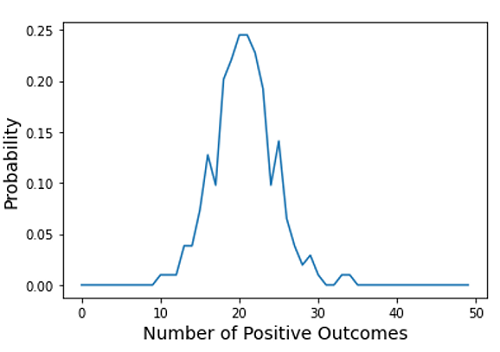# Python | Binomial Experiment Simulation

Python | Binomial Experiment Simulation: In this tutorial, we are going to learn about the binomial experiment simulation and its python implementation.
Submitted by Anuj Singh, on July 09, 2020

A binomial experiment is described by the following characteristics:

• An experiment that involves repeated trials.
• Each trial can only have two possible outcomes i.e. success or failure.
• The likelihood of a particular outcome will occur on any given trial that remains constant throughout the experiment.
• All of the trials are independent of the experiment.

An experiment of a series of coin tosses is a perfect example of a binomial experiment. In this article, we are going to simulate a binomial experiment using an inbuilt function numpy.random.binomial(). This NumPy library function returns a vector containing the number of positive outcomes in n number of trials.

Probability of positive outcome: 0.2

Following is the python code for demonstration and plotting Probability Distribution of Simulation Binomial Process:

```import numpy as np
import matplotlib.pyplot as plt

n = 100
p = 0.2
x = np.random.binomial(n,p,size = 19)

kk = 0
kr = []
l = 0

for i in range(50):
n = 100
kk = 0
for h in range(100):
# j.append(np.random.binomial(i,p))
jj = np.random.binomial(n,p)
if jj >= i:
kk = kk + 1
kr.append(kk*(100-kk)/10000)
# it is number of head
# find the probability of number of head

print(kr)

plt.plot(kr)
plt.xlabel('Number of Positive Outcomes',fontsize=14)
plt.ylabel('Probability',fontsize=14)
```

Output:Preparation

What's New

Top Interview Coding Problems/Challenges!